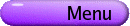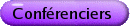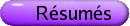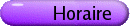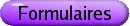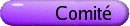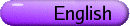Topologie géométrique Org: Hans Boden (McMaster), B. Doug Park (Waterloo) et Mainak Poddar (Waterloo)[PDF] TARA BRENDLE, Cornell University, Malott Hall, Ithaca, NY 14853 The Birman-Craggs-Johnson homomorphism and the cohomology of the Torelli group [PDF] The Torelli group Tg is the subgroup of the mapping class group of a surface consisting of those diffeomorphism classes acting trivially on the homology of the surface. The Torelli group is the "mysterious part" of the mapping class group, and basic questions about its structure (e.g., finite presentability) are still not known; in particular its homology is known only in dimension one. In the 1970/80s Birman-Craggs-Johnson constructed a remarkable surjective homomorphism from Tg to a certain Z/2Z-vector space of Boolean (square-free) polynomials. The heart of this construction comes from the Rochlin invariant for homology 3-spheres. This homomorphism, together with abelian cycles in Tg, can be used to construct nontrivial elements of H2(Tg, Z/2Z), which had not been detected rationally. This construction yields a lower bound on the order of g4 for the rank of this homology group. We will also discuss the question of lifting these classes to integral classes and the connection with the "Casson-Morita algebra". This is joint work in progress with Benson Farb. OLIVIER COLLIN, Université du Québec à Montréal Floer homology of links of complex singularities and analytical invariants of Milnor fibres [PDF] In this talk, we survey some aspects of Floer homology for homology spheres and for knots that are relevant for the study of analytical invariants of Milnor fibres of 3-manifolds that arise as links of complex singularities. MARIANTY IONEL, McMaster University, Hamilton Special Lagrangian submanifolds in the cotangent bundle of the sphere [PDF] The cotangent bundle of the sphere T* Sn has a Ricci-flat Kahler metric discovered by Stenzel. The case n=1 is the Eguchi-Hanson metric and the case n=2 was found earlier by Candelas and de la Ossa. In this talk I will present examples of cohomogeneity one special Lagrangian 3-folds in the case n=3. Some of these examples can be generalized for any dimension of the sphere. We will also address the asymptotic behaviour of these submanifolds and their topology. This is work in progress with M. Min-Oo. ERNESTO LUPERCIO, Cinvestav-IPN (Center for Research and Advanced Studies, Mexico) Orbifold String Topology [PDF] In this talk I will introduce first the string topology product of Chas and Sullivan on the homology of the free loop space of a manifold LM. Then I will explain the Cohen-Jones theory that gives a homotopy theory interpretation of the product and finally I will close by explaining our construction of the string topology ring for a orbifold (real differentiable smooth Deligne-Mumford Stack). I will hint at the relation to the Chen-Ruan orbifold cohomology product, and its relations to non-commutative geometry. This is a report of my work in progress with B. Uribe and M. A. Xicotencatl. JOSEPH MASTERS, SUNY Buffalo Quasi-Fuchsian surfaces in hyperbolic knot manifolds [PDF] We show that every 1-cusp, finite-volume hyperbolic 3-manifold admits an essential, quasi-Fuchsian immersion of a closed, hyperbolic surface. Joint work with Xingru Zhang. BRENDAN OWENS, Cornell University, Ithaca, NY 14853 Unknotting information from Heegaard Floer homology [PDF] I will describe Ozsvath-Szabo's obstruction to a knot having unknotting number one, and a generalisation to higher unknotting numbers. This leads to the completion of the table of unknotting numbers for prime knots with 9 crossings or less. STEPHAN TILLMANN, Université du Québec à Montréal Angle structures and geometric splittings [PDF] The study of angle structures on an ideally triangulated 3-manifold (M,T) goes back to Casson, who noticed that the existence of such a "linear hyperbolic" structure has strong topological consequences: M is irreducible, atoroidal and its ends are tori or Klein bottles. In this talk I describe topological consequences in the case where a degenerate angle structure (some angles may be 0) is dual to a normal surface. The surface turns out to be essential, and the associated splitting of a uniquely associated Dehn-Thurston surgery of M is geometric: the pieces are Seifert fibered spaces or "linear hyperbolic" cone-manifolds. I will also explain how this illustrates the Culler-Shalen machine in special cases. STEFANO VIDUSSI, Department of Mathematics, 138 Cardwell Hall, Kansas State University, Manhattan, KS 66506 Alexander polynomials and symplectic S1 xN [PDF] We will present some results and speculations concerning the connection between the study of the Alexander polynomials of a three manifold N and the problem of determining when S1 ×N admits a symplectic structure. MEI-LIN YAU, Michigan State University, East Lansing, MI 48824, USA A holomorphic 0-surgery model with application to cylindrical contact homology [PDF] It is known that a contact structure on a closed 3-manifold M is supported by an open book representation of M. By applying 0-surgery to every connected component of of the binding one gets a new manifold M¢ which is a mapping torus of a closed surface. Both RxM and RxM¢ can be endowed with R-invariant symplectic structures as well as R-invariant compatible almost complex structures. In this talk we give, in the complex plane, a simple holomorphic model of the 0-surgery. This model allows explicit relations between pseudoholomorphic curves in RxM and pseudoholomorphic curves in RxM¢. As an application, we use it to compute the cylindrical contact homology of open books resulting from a positive Dehn twist on a torus with boundary. These are the first examples of cylindrical contact homology via open books with nontrivial monodromy.